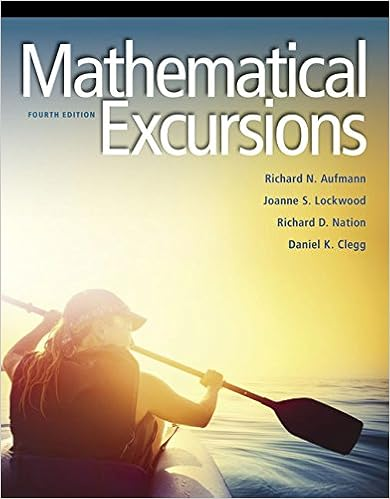# Catherine chao director of marketing research needs a

• Test Prep
• 9
• 100% (1) 1 out of 1 people found this document helpful

This preview shows page 7 - 9 out of 9 pages.

Test 2 Ch 5-7 (1).rtf

Catherine Chao Director of Marketing Research needs a sample of households to from MS 1023 at University of Texas, San Antonio

27 .   On Saturdays , cars arrive at David Zebda 's Scrub and Shine Car Wash at the rate of 80 cars per hour during the ten - hour shift . David wants a sample of 40 Saturday customers to answer the long version of his quality service questionnaire . He randomly selects 9 as a starting point and instructs the crew to select the 9th customer and each customer at intervals of 20 ( 9 , 29 , 49 , etc. ) . His sample is a __________.
A ) convenience sample
B ) simple random sample
C ) unsystematic sample
D ) stratified sample
E ) systematic sample
28 .   Catherine Chao , Director of Marketing Research , needs a sample of households to participate in the testing of a new toothpaste package . She directs the seven members of her staff to find five households each . Catherine 's sample is a __ _ _ _ ________.
29 .   The average age of a UTSA student is 25.6 years with a standard deviation of 8.3 years . If a random sample of 45 students were taken in a classroom with 300 students , what is the probability that the sample will have an average age of less than 28 years ?
30 .   According to the central limit theorem , if a sample of size 56 is drawn from a population with a standard deviation of 14 , the standard error of the distribution of the sample means would equal _ ______.
31 .   Suppose the population of all public Universities shows the annual parking fee per student is $110 with a standard deviation of$ 18 . If a random sample of size 49 is drawn from the population , the probability of drawing a sample with a mean of more than $115 is _______. A ) 0.9738 B ) 0.4738 C ) 0.0262 D ) 0.3897 E ) 0.1103 32 . Suppose the population of all public Universities shows the annual parking fee per student is$ 110 with a standard deviation of $18 . If a random sample of size 49 is drawn from the population , the probability of drawing a sample with a mean of less than$ 92 is _ ______.
33 .   Suppose the population of all public Universities shows the annual parking fee per student is $110 with a standard deviation of$ 18 . If a random sample of size 49 is drawn from the population , the probability of drawing a sample with a sample mean between $100 and$ 115 is _______.
34 .   Suppose 40 % of the population of pre-teens have a TV in their bedroom . If a random sample of 500 pre-teens is drawn from the population , then the probability that 44 % or fewer of the pre-teens have a TV in their bedroom is _______.
##### We have textbook solutions for you!
The document you are viewing contains questions related to this textbook.The document you are viewing contains questions related to this textbook.
Chapter 13 / Exercise 46
Mathematical Excursions
AufmannExpert Verified
28.Catherine Chao, Director of Marketing Research, needs a sample of households to participate in the testing of a new toothpaste package. She directs the seven members of her staff to find five households each. Catherine's sample is a _____________.A)cluster sampleB)proportionate stratified sampleC)quota sampleD)disproportionate stratified sampleE)simple random sample
29.The average age of a UTSA student is 25.6 years with a standard deviation of 8.3 years. If a random sample of 45 students were taken in a classroom with 300 students, what is the probability that the sample will have an average age of less than 28 years?
30.According to the central limit theorem, if a sample of size 56 is drawn from a population with a standard deviation of 14, the standard error of the distribution of the sample meanswould equal _______.
Page 7
##### We have textbook solutions for you!
The document you are viewing contains questions related to this textbook.The document you are viewing contains questions related to this textbook.
Chapter 13 / Exercise 46
Mathematical Excursions
AufmannExpert Verified
31.Suppose the population of all public Universities shows the annual parking fee per student is $110 with a standard deviation of$18. If a random sample of size 49 is drawn from the population, the probability of drawing a sample with a mean of more than $115 is _______. 32.Suppose the population of all public Universities shows the annual parking fee per student is$110 with a standard deviation of $18. If a random sample of size 49 is drawn from the population, the probability of drawing a sample with a mean of less than$92 is _______.A)0.3400B)0.1600C)0.0000D)1.0000E)0.7000
33.Suppose the population of all public Universities shows the annual parking fee per student is $110 with a standard deviation of$18. If a random sample of size 49 is drawn from the population, the probability of drawing a sample with a sample mean between $100 and$115 is _______.
34.Suppose 40% of the population of pre-teens have a TV in their bedroom. If a random sample of 500 pre-teens is drawn from the population, then the probability that 44% or fewer of the pre-teens have a TV in their bedroom is _______.
Page 8
Page 9
•••